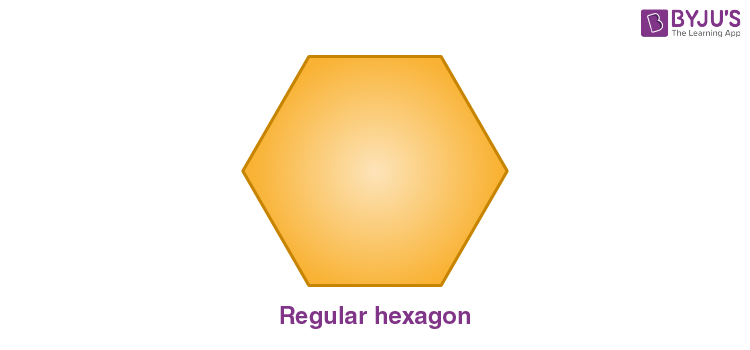# Regular Hexagon

A regular hexagon is a closed shape polygon which has six equal sides and six equal angles. In case of any regular polygon, all its sides and angles are equal. For instance, a regular pentagon has 5 equal sides and a regular octagon has 8 equal sides. When such conditions are not met, polygons may resemble a variety of irregular shapes. When we arrange six equilateral triangles side by side, then a regular hexagon is composed. Then, the area of the regular hexagon becomes equal to six times the area of the same triangle. Let us learn its properties, formula for area, perimeter in this article.## Regular Hexagon Properties

• It has 6 equal sides and 6 equal angles.
• It has 6 vertices.
• Sum of interior angles equals 720°.
• Interior angle is 120° and exterior angle is 60°.
• It is made up of six equilateral triangles.
• 9 diagonals can be drawn inside a regular hexagon.
• All the sides opposite to each other are parallel.

## Area and Perimeter of Regular Hexagon

We have thrown light on the definition and properties of regular hexagon till now, let us learn about its area and perimeter.

Area of Regular Polygon is given by;

 A = 3√3/2 × a2 = 2.59807 a2

Where a is the measurement of its sides or length of the side.

Perimeter of Hexagon is equal to;

 P = 6a

where a is the side length.

## Angles of Regular Hexagon

As we already know, the sum of the angles of the regular hexagon which has all its 6 sides equal is 720°. Therefore, it’s interior angle and exterior angle is given by;

Each interior angle = 720°/6 = 120°

Each exterior angle = 180°- interior angle = 180°-120° = 60°

### Examples

Q.1: Find the area and perimeter of hexagon, if all its sides have a length equal to 6cm.

Solution: From the area and perimeter formula of a regular hexagon, we know;

Area = 2.59807 a2 = 2.59807 × 62 = 93.53 cm2

Perimeter = 6a = 6 × 6 = 36 cm

Q.2: Evaluate the length of the side of a regular hexagon if its perimeter is given as 48cm.

Solution: Given, perimeter = 48cm

By the formula, we know;

Perimeter = 6a

Therefore,

48 = 6a

Or

a = 48/6 = 8cm

Hence the length of the side to given hexagon is 8cm

Test your Knowledge on Regular Hexagon